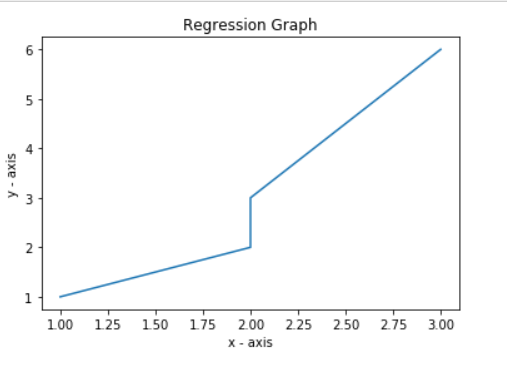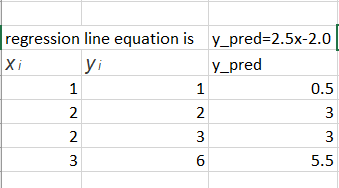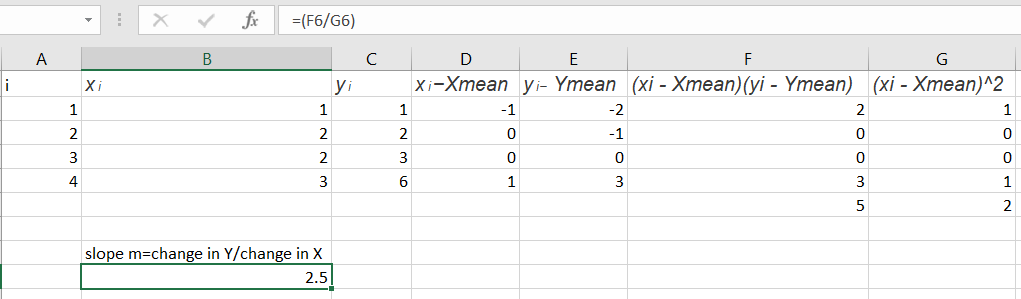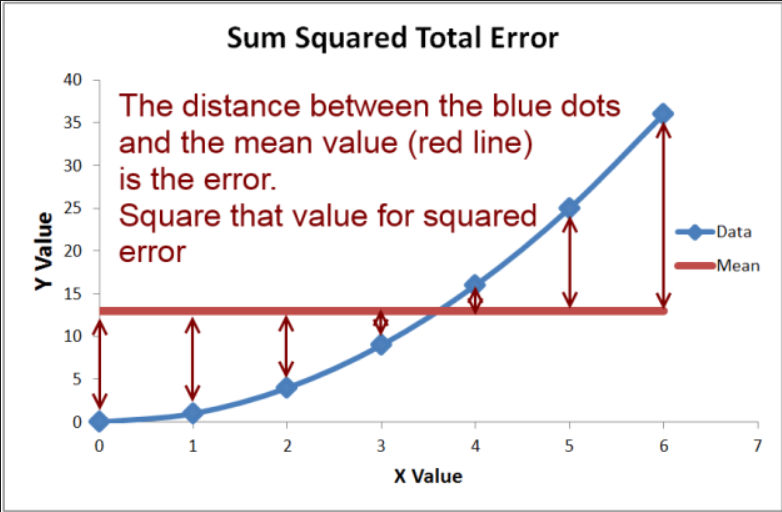# ML | Mathematical explanation of RMSE and R-squared error

RMSE: Root Mean Square Error is the measure of how well a regression line fits the data points. RMSE can also be construed as Standard Deviation in the residuals.
Consider the given data points: (1, 1), (2, 2), (2, 3), (3, 6).
Lets break the above data points into 1-d lists.
Input :

```x = [1, 2, 2, 3]
y = [1, 2, 3, 6]```

Code : Regression Graph

 `import` `matplotlib.pyplot as plt  ` `import` `math ` ` `  `# plotting the points   ` `plt.plot(x, y)  ` ` `  `# naming the x axis  ` `plt.xlabel(``'x - axis'``)  ` ` `  `# naming the y axis  ` `plt.ylabel(``'y - axis'``)  ` ` `  `# giving a title to my graph  ` `plt.title(``'Regression Graph'``)  ` ` `  `# function to show the plot  ` `plt.show()  `Code: Mean Calculation

 `# in the next step we will find the equation of the best fit line ` `# we will use Linear algebra's Point slope form to find regression line equation ` `# point-slope form is represented by y = mx + c ` `# where m is slope means (change in y) / (change in x) ` `# c is constant, it represents at which point line will intercept y-axis ` `# slope m can be formulated as below: ` `''' ` `   ``n ` `m =? (xi - Xmean) (yi - Ymean)/?(xi - Xmean)^2 ` ` ``i = 1 ` `'''` `# calculate Xmean and Ymean ` `ct ``=` `len``(x) ` `sum_x ``=` `0` `sum_y ``=` `0` ` `  `for` `i ``in` `x: ` `    ``sum_x ``=` `sum_x ``+` `i ` `x_mean ``=` `sum_x ``/` `ct ` `print``(``'Value of X mean'``, x_mean) ` ` `  `for` `i ``in` `y: ` `    ``sum_y ``=` `sum_y ``+` `i ` `y_mean ``=` `sum_y ``/` `ct ` `print``(``'value of Y mean'``, y_mean) ` ` `  `# we have the values of x mean and y_mean `

Output :

```Value of X mean 2.0
value of Y mean 3.0
```

Code : Line Equation

 `# below is the process of finding line equation in mathematical terms ` `# slope of our line is 2.5 ` `# calculate c to find out the equation ` ` `  `m ``=` `2.5` `c ``=` `y_mean ``-` `m ``*` `x_mean ` `print``(``'Intercept'``, c) `

Output :

`Intercept -2.0`

Code : Mean Squared Error

 `# equation of our Regression line comes out to be as below: ` `# y_pred = 2.5x-2.0 ` `# we call the line y_pred ` `# paste regression line graph ` `from` `sklearn.metrics ``import` `mean_squared_error  ` `# y_pred for our exusting data points is as below ` ` `  `y ``=``[``1``, ``2``, ``3``, ``6``] ` `y_pred ``=``[``0.5``, ``3``, ``3``, ``5.5``] ``# root mean square calculated by sklearn package ` `mse ``=` `math.sqrt(mean_squared_error(y, y_pred)) ` `print``(``'Root mean square error'``, mse) `

Output :

```Root mean square error 0.6123724356957945
```

Code : RMSE Calculation

 `# lets check how the Root mean square is calculated mathematically ` `# lets introduce a term called residuals ` `# residual are basically the distance of data point from the regression line ` `# residuals are denoted by red marked line in below graph ` `# root mean square and residuals are calculated as below ` `# we have 4 data points  ` `''' ` `r = 1, ri = yi-y_pred ` `y_pred is mx + c ` `ri = yi-(mx + c)  ` `e.g. x = 1, we have value of y as 1 ` `we want to evaluate what exactly our model has predicted for x = 1 ` `(1, 1)r1 = 1, x = 2  ` `'''` `# y_pred1 = 1-(2.5 * 1-2.0)= 0.5 ` `r1 ``=` `1``-``(``2.5` `*` `1``-``2.0``) ` ` `  `#(2, 2) r2 = 2, x = 2  ` `# y_pred2 = 2-(2.5 * 2-2.0)=-1 ` `r2 ``=` `2``-``(``2.5` `*` `2``-``2.0``) ` ` `  `#(2, 3) r3 = 3, x = 2  ` `# y_pred3 = 3-(2.5 * 2-2.0)= 0 ` `r3 ``=` `3``-``(``2.5` `*` `2``-``2.0``) ` ` `  `#(3, 6) r4 = 4, x = 3  ` `# y_pred4 = 6-(2.5 * 3-2.0)=.5 ` `r4 ``=` `6``-``(``2.5` `*` `3``-``2.0``) ` ` `  `# from above calculation we have values of residuals ` `residuals ``=``[``0.5``, ``-``1``, ``0``, .``5``] ` ` `  `# now calculate root mean square error ` `# N = 4 data points ` `N ``=` `4` `rmse ``=` `math.sqrt((r1``*``*``2` `+` `r2``*``*``2` `+` `r3``*``*``2` `+` `r4``*``*``2``)``/``N) ` `print``(``'Root Mean square error using maths'``, rmse) ` ` `  `# root mean square actually calculated using mathematics ` `# both of RMSE calculated are same `Output :

`Root Mean square error using maths 0.6123724356957945`

R-squared Error or Coefficient of Determination
R2 error answers the below question.
How much y varies with variation in x.Basically the % variation of y on variation with xCode : R-Squared Error

 `# SEline =(y1-(mx1 + b)**2 + y2-(mx2 + b)**2...+yn-(mxn + b)**2) ` `# SE_line =(1-(2.5 * 1+(-2))**2 + (2-(2.5 * 2+(-2))**2) +(3-(2.5*(2)+(-2))**2) + (6-(2.5*(3)+(-2))**2)) ` ` `  `val1 ``=``(``1``-``(``2.5` `*` `1``+``(``-``2``)))``*``*``2` `val2 ``=``(``2``-``(``2.5` `*` `2``+``(``-``2``)))``*``*``2` `val3 ``=``(``3``-``(``2.5` `*` `2``+``(``-``2``)))``*``*``2` `val4 ``=``(``6``-``(``2.5` `*` `3``+``(``-``2``)))``*``*``2` `SE_line ``=` `val1 ``+` `val2 ``+` `val3 ``+` `val4 ` `print``(``'val'``, val1, val2, val3, val4) ` ` `  `# next to calculate total variation in Y from mean value ` `# variation in y is calcualted as ` `# y_var =(y1-ymean)**2+(y2-ymean)**2...+(yn-ymean)2 ` ` `  `y ``=``[``1``, ``2``, ``3``, ``6``] ` ` `  `y_var ``=``(``1``-``3``)``*``*``2``+``(``2``-``3``)``*``*``2``+``(``3``-``3``)``*``*``2``+``(``6``-``3``)``*``*``2` `SE_mean ``=` `y_var ` ` `  `# by calculating y_var we are calculating the distance ` `# between y data points and mean value of y ` `# so answer to our question, % of the total variation ` `# of wrt x is denoted as below: ` `r_squared ``=` `1``-``(SE_line ``/` `SE_mean) ` ` `  `# [SE_line / SE_mean] -->tells us the what % of variation ` `# in y is not described by regression line ` `# 1-(SE_line / SE_mean) --> gives us the exact value of  ` `# how much % y varies with variation in x ` `print``(``'Rsquared error'``, r_squared) `

Output :

```Rsquared error 0.8928571428571429
```

Code : R-Squared Error with sklearn

 `from` `sklearn.metrics ``import` `r2_score ` ` `  `# r2 error calculated by sklearn is similar  ` `# to ours mathematically calculated r2 error ` `# calculate r2 error using sklearn ` `r2_score(y, y_pred) `

Output :

`0.8928571428571429`

My Personal Notes arrow_drop_upCheck out this Author's contributed articles.

If you like GeeksforGeeks and would like to contribute, you can also write an article using contribute.geeksforgeeks.org or mail your article to contribute@geeksforgeeks.org. See your article appearing on the GeeksforGeeks main page and help other Geeks.

Please Improve this article if you find anything incorrect by clicking on the "Improve Article" button below.

Article Tags :
Practice Tags :

4

Please write to us at contribute@geeksforgeeks.org to report any issue with the above content.